# Top-open tank

The top-open tank has the shape of a truncated rotating cone, which stands on a smaller base. The tank's volume is 465 m3, the radii of the bases are 4 m and 3 m. Find the depth of the tank.

h =  12 m

### Step-by-step explanation: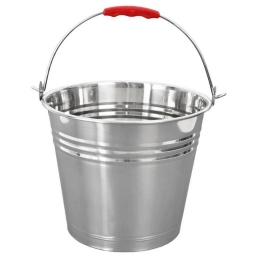Did you find an error or inaccuracy? Feel free to write us. Thank you!Tips to related online calculators
Tip: Our volume units converter will help you with the conversion of volume units.

#### You need to know the following knowledge to solve this word math problem:

We encourage you to watch this tutorial video on this math problem:

## Related math problems and questions:

• The truncatedThe truncated rotating cone has bases with radii r1 = 8 cm, r2 = 4 cm and height v = 5 cm. What is the volume of the cone from which the truncated cone originated?
• Truncated cone 3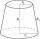The surface of the truncated rotating cone S = 7697 meters square, the substructure diameter is 56m and 42m, find the height of the tang.
• Truncated coneCalculate the height of the rotating truncated cone with volume V = 794 cm3 and a base radii r1 = 9.9 cm and r2 = 9.8 cm.
• Frustrum - volume, area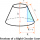Calculate the surface and volume of a truncated rotating cone with base radii of 8 cm and 4 cm, height 5 cm.
• Truncated cone 6Calculate the volume of the truncated cone whose bases consist of an inscribed circle and a circle circumscribed to the opposite sides of the cube with the edge length a=1.
• Frustrum - volume, areaCalculate the surface and volume of the truncated cone, the radius of the smaller figure is 4 cm, the height of the cone is 4 cm and the side of the truncated cone is 5 cm.
• Frustum of a cone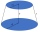A reservoir contains 28.54 m3 of water when full. The diameter of the upper base is 3.5 m, while at the lower base is 2.5 m. Find the height if the reservoir is in the form of a frustum of a right circular cone.
• The surfaceThe surface of a truncated rotating cone with side s = 13 cm is S = 510π cm2. Find the radii of the bases when their difference in lengths is 10cm.
• Truncated pyramidHow many cubic meters is the volume of a regular four-sided truncated pyramid with edges one meter and 60 cm and high 250 mm?
• Rotating coneFind the rotating cone's surface and volume if its side is 150 mm long and the circumference of the base is 43.96 cm.
• Digging a pit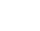The pit has the shape of a regular quadrilateral truncated pyramid. The edges of the bases are 14m and 10m long. The sidewalls form an angle of 135° with a smaller base. Determine how many m3 of soil were excavated when digging the pit?
• Cone - from volume surface areaThe volume of the rotating cone is 1,018.87 dm3, and its height is 120 cm. What is the surface area of the cone?
• Base of prismThe base of the perpendicular prism is a rectangular triangle whose legs length are at a 3: 4 ratio. The height of the prism is 2cm smaller than the larger base leg. Determine the volume of the prism if its surface is 468 cm2.
• Slant heightThe cone's slant height is 5cm, and the radius of its base is 3cm, find the volume of the cone.
• Truncated coneA truncated cone has bases with radiuses of 40 cm and 10 cm and a height of 25 cm. Calculate its surface area and volume.
• Cuboid edges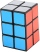The lengths of the cuboid edges are in the ratio 2: 3: 4. Find their length if you know that the surface of the cuboid is 468 m2.
• Prism basesVolume perpendicular quadrilateral prism is 360 cm3. The edges of the base and height of the prism are in the ratio 5:4:2. Determine the area of the base and walls of the prism.